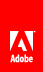# decimal

Holds a decimal value.

In the AEM forms database, values are represented by an unscaled integer value and a scale that determines the location of the decimal. The scale attribute indicates the number of digits in the integer that are on the right side of the decimal. The default scale is 3 .

For example, an integer value of 12345 and a scale of 3 represents the decimal value of 12.345 .

The length attribute determines the maximum number of digits that can be stored. The default length is 10 .

You can change the values of the scale and length attributes using the Variables view.

A decimal number

#### Example

`123.4`// Ethnio survey code removed